2021 NFL Offensive Rookie of the Year Odds
+110
1.1 to 1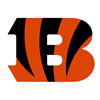Ja'Marr Chase
30.5% implied probability

+400
4 to 1Mac Jones
12.8% implied probability

+800
8 to 1Najee Harris
7.1% implied probability

+1000
10 to 1Trevor Lawrence
5.8% implied probability

+1400
14 to 1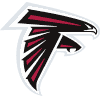Kyle Pitts
4.3% implied probability

+1600
16 to 1Trey Lance
3.8% implied probability

+2000
20 to 1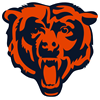Justin Fields
3.1% implied probability

+3500
35 to 1Elijah Mitchell
1.8% implied probability

+3500
35 to 1Rondale Moore
1.8% implied probability

+4000
40 to 1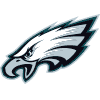Kenneth Gainwell
1.6% implied probability

+4000
40 to 1Javonte Williams
1.6% implied probability

+4000
40 to 1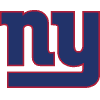Kadarius Toney
1.6% implied probability

+4000
40 to 1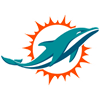Jaylen Waddle
1.6% implied probability

+5000
50 to 1Chuba Hubbard
1.3% implied probability

No changes have been recorded yet.
+5000
50 to 1Zach Wilson
1.3% implied probability

+5000
50 to 1DeVonta Smith
1.3% implied probability

+6000
60 to 1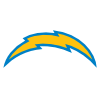Rashawn Slater
1.1% implied probability

+6000
60 to 1Rashod Bateman
1.1% implied probability

+6500
65 to 1Michael Carter
1.0% implied probability

+8000
80 to 1Trey Sermon
0.8% implied probability

+10000
100 to 1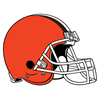Anthony Schwartz
0.6% implied probability

+10000
100 to 1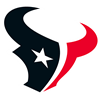Davis Mills
0.6% implied probability

+10000
100 to 1Alijah Vera-Tucker
0.6% implied probability

+10000
100 to 1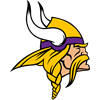Kellen Mond
0.6% implied probability

+10000
100 to 1Josh Palmer
0.6% implied probability

+10000
100 to 1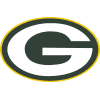Amari Rodgers
0.6% implied probability

+10000
100 to 1Cade Johnson
0.6% implied probability

+10000
100 to 1Tommy Tremble
0.6% implied probability

+10000
100 to 1Brevin Jordan
0.6% implied probability

+10000
100 to 1Kyle Trask
0.6% implied probability

+10000
100 to 1Tylan Wallace
0.6% implied probability

+10000
100 to 1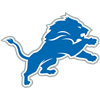Amon-Ra St. Brown
0.6% implied probability

+10000
100 to 1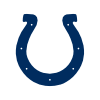Sam Ehlinger
0.6% implied probability

+10000
100 to 1Chris Evans
0.6% implied probability

+10000
100 to 1Khalil Herbert
0.6% implied probability

+10000
100 to 1Dyami Brown
0.6% implied probability

+10000
100 to 1Pat Freiermuth
0.6% implied probability

+10000
100 to 1Hunter Long
0.6% implied probability

+10000
100 to 1Jaelon Darden
0.6% implied probability

+10000
100 to 1Terrace Marshall
0.6% implied probability

+10000
100 to 1Elijah Moore
0.6% implied probability

+10000
100 to 1Penei Sewell
0.6% implied probability

+10000
100 to 1Jamie Newman
0.6% implied probability

+10000
100 to 1Tutu Atwell
0.6% implied probability